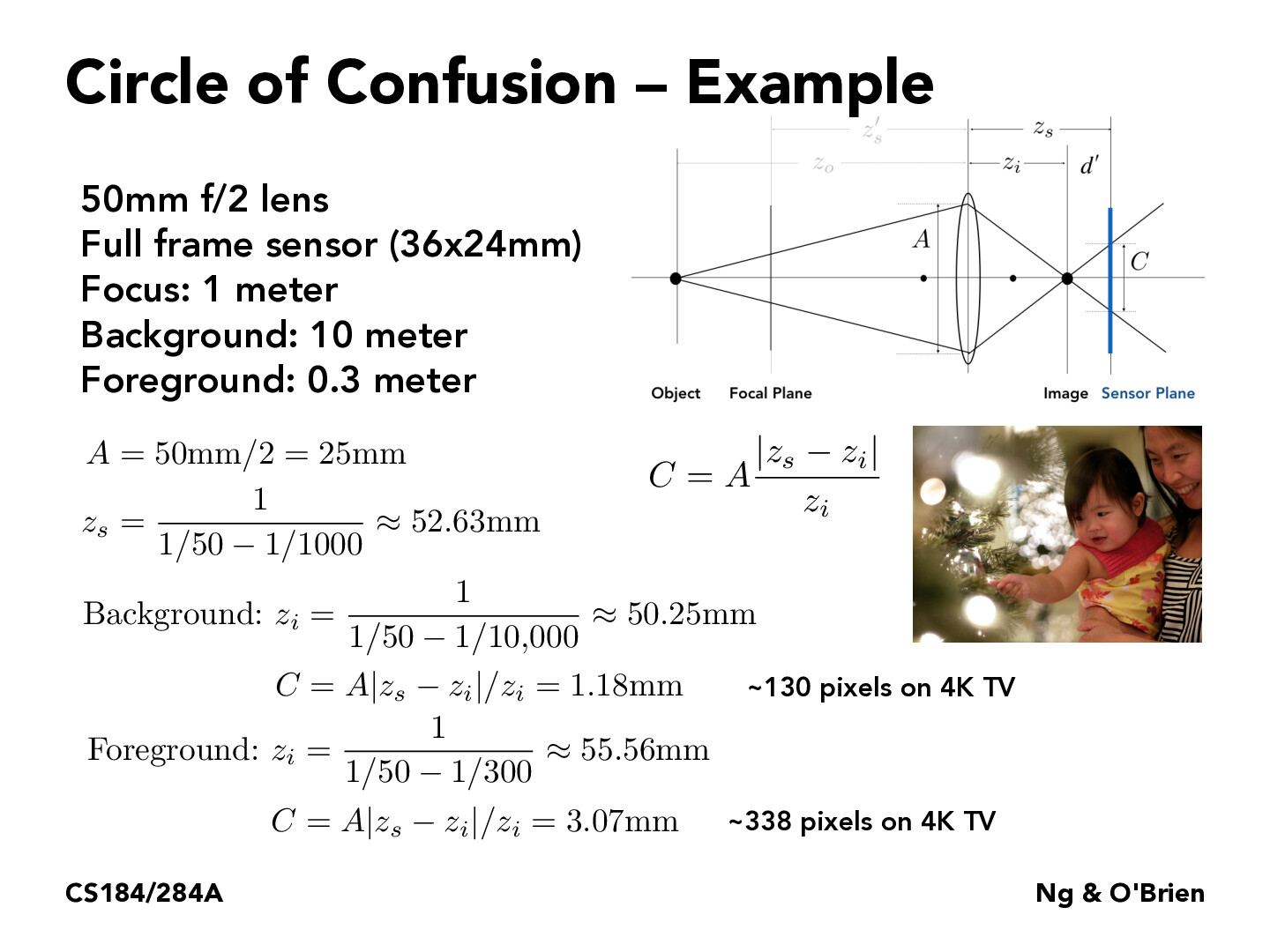Lecture 15: Cameras & Lenses (88)mylinhvu11

To clarify, based on my observations, the values for the z values follow the equation: 1 / ((1 / lens length) - (1 / focus length of respective z value)). Where does this equation come from and what exactly do these z values signify? the distances from the lens?degaliang

What is the significance of the C values here? Why do we care about it?daniel-man

@degaliang The C values are significant as they represent the diameter of the circle of confusion, where a cone of light rays coming from a lens do not converge to a perfect focus, instead creating a blurred circular shape. We care about this diameter value because a larger diameter is indicative of a closer defocused point light, while a smaller diameter is indicative of a further defocused point light.Staffyirenng

@mylinhvu11 -- $z_i$ is the distance between the lens and optical image of the object in the world. The equations for $z_i$ here come directly from the thin lens equation on the previous slides, rearranging to solve for $z_i$ as a function of $f$ and $z_o$. In this problem, we are given that $f$ = 50mm is the focal length of the lens, and we are analyzing the circles of confusion for two objects in the world -- lights in the foreground and lights in the background. For the lights in the foreground, $z_o$ = 0.3m = 300mm is the distance from the lens to those lights. For the lights in the background, $z_o$ = 10m = 10,000mm.

Does this help?Staffyirenng

@daniel-man -- thanks for responding to #degaliang, and you're right that $C$ represents the diameter of the circle of confusion.

However, I should caution that a larger $C$ will not always indicate that the defocused object is closer than the world focal plane. That is just the situation in this example photo. You can convince yourself of this by looking at the equations, and reasoning about what would happen if the foreground lights were closer to the focal plane -- $C$ will decrease. The intuition is that the lights will be less blurred and have smaller $C$, the closer they are to the plane of focus.sZwX74

I think the "closer" and "further" actually being "closer to the focal plane" and "further from the focal plane" can also be geometrically explained by looking at the diagram, and considering what would happen if we moved the object to the other side of the focal plane but the same distance away. In that case, if we sort of "extend" the light rays from the object in front to where they hit the focal plane, then it would strike the same range, and therefore the light rays would strike that same range on the sensor plane, but the focus image would converge behind it instead. I think this helps explain the symmetrical behavior about the plane of focus, and why it is not just "closer" or "farther" from the lens itself.stexus

What exactly dictates $z_s$? Isn't it effectively continuous so there are circles of confusion basically everywhere? Or are they specifically only light sources? It's a little confusing because any distance away where there is light we can consider that a pseudo light source mathematically from just the rays coming from it.

You must be enrolled in the course to comment